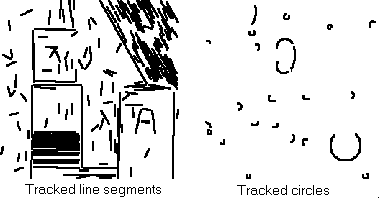Edge tracking and fitting
of line and arc segmentsAs a further example, we show an approach which tracks edge data in a local neighbourhood, yet fits straight line and circular arc segments as it proceeds. It is a three pass algorithm, which operates on thinned edge data. The algorithm is not described in detail; a description can be found in ``Robust segmentation of edge data'' in the Proc. of the 4th IEE International Conf. on Image Processing, 1992, written by A. Etemadi.

• Segmentation Pass: the usual way to segment a chain of pixels is by examination of curvature. This algorithm proceeds in a slightly diferent manner; the chained sets of pixels are broken into subsets which are near-symmetric about an axis passing through the midpoint of the subset, but perpendicular to the line joining the end points.
• Linking Adjacent Segments: a least squares fit is performed on segment points. If adjacent segments are to be merged, then they must have the same relative direction and radius of curvature, to within a specified tolerance. A new fit may be performed on the linked data and the process repeated.
• Classification: this is done by a simple heuristic, checking the deviation of the central point from a straight line joining the end points. Segments are classified as straight or circular.

Figures 5 and 6, below, show an example of the algorithm applied to a test image. Here, line or arcs of less than 10 pixels are rejected.Figure 5: The test imageFigure 6: Edge tracking and fittingComments to: Sarah Price at ICBL.
(Last update: 22th April, 1996)